﻿ Pythagorean theorem stock photo. Image of billboard, professor - 27393326

# Pythagorean theorem.

## Pythagorean theorem sketched with white chalk on a chalk board

Designers also selected these stock photosHighway interchange.Dealing With DementiaCrowing a PrincessBlaise Pascal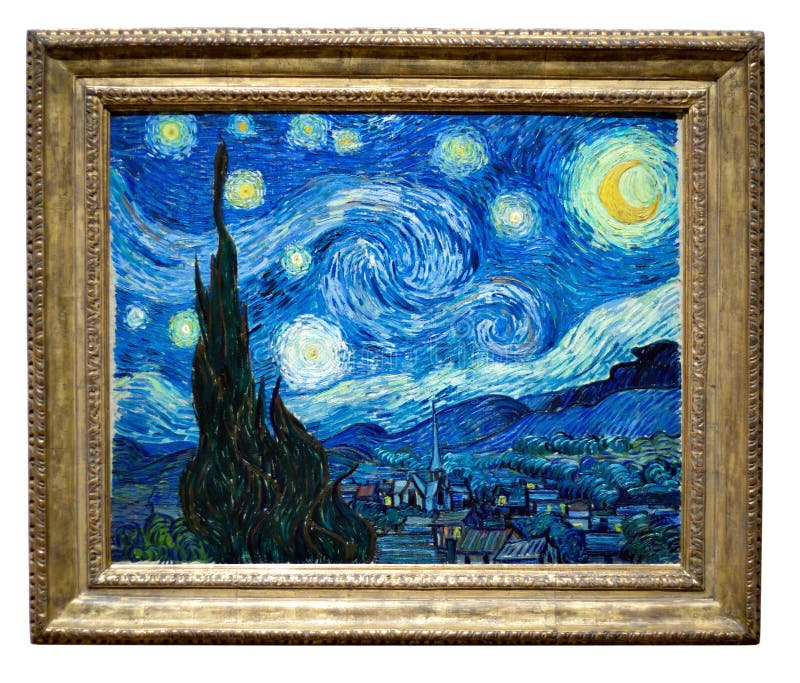Starry Night Painting By VincentPeople at retail shopping mallKnight in heavy armor on a horse and with a lanceCute guy on beachgirl model. Beautiful face of young adult woman with clean
More similar stock images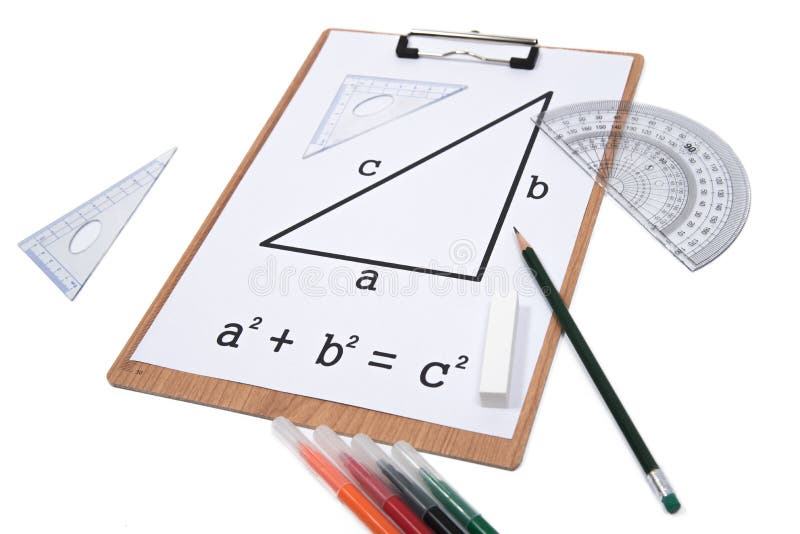Pythagorean Theorem.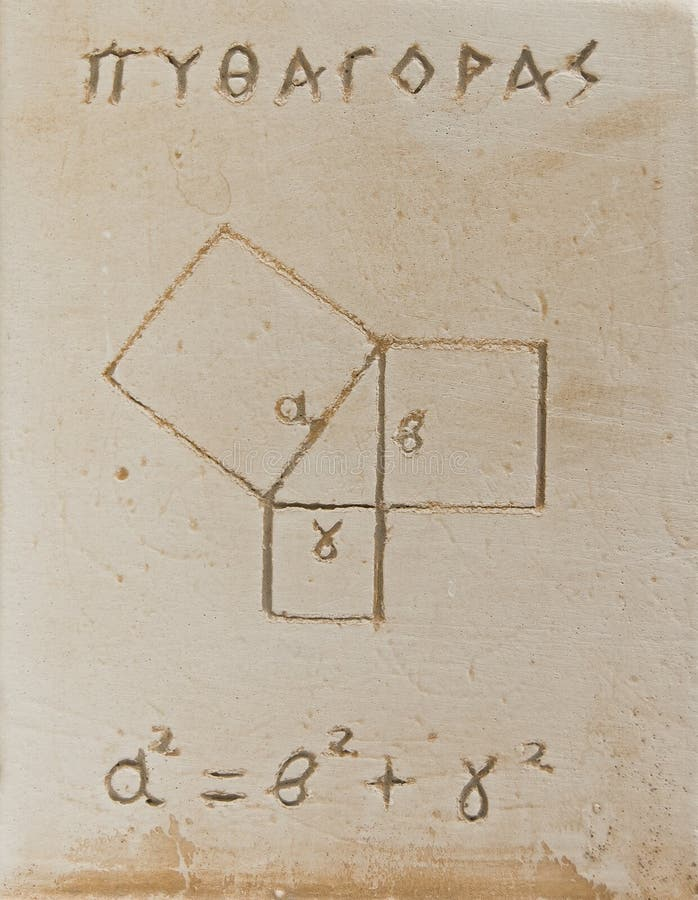Pythagorean theorem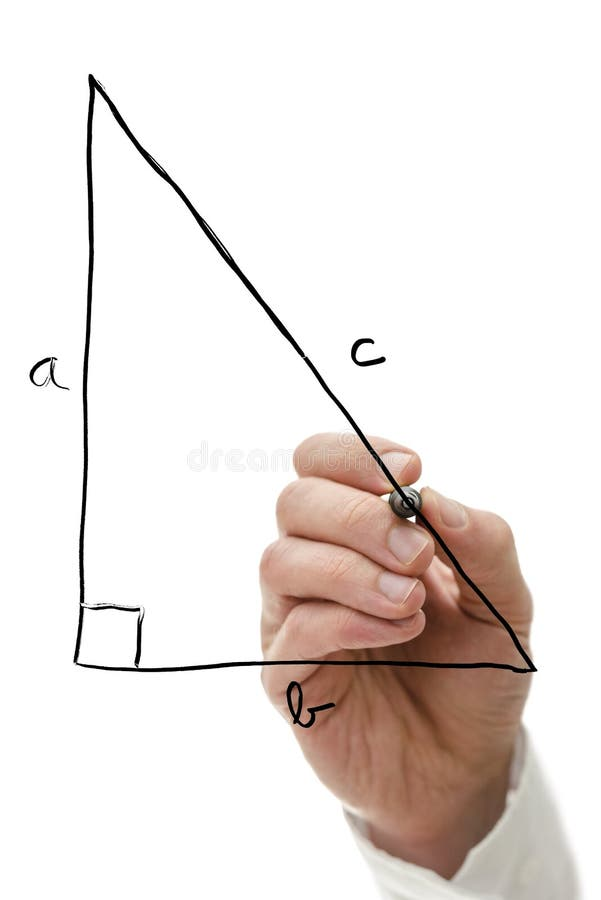Pythagorean theorem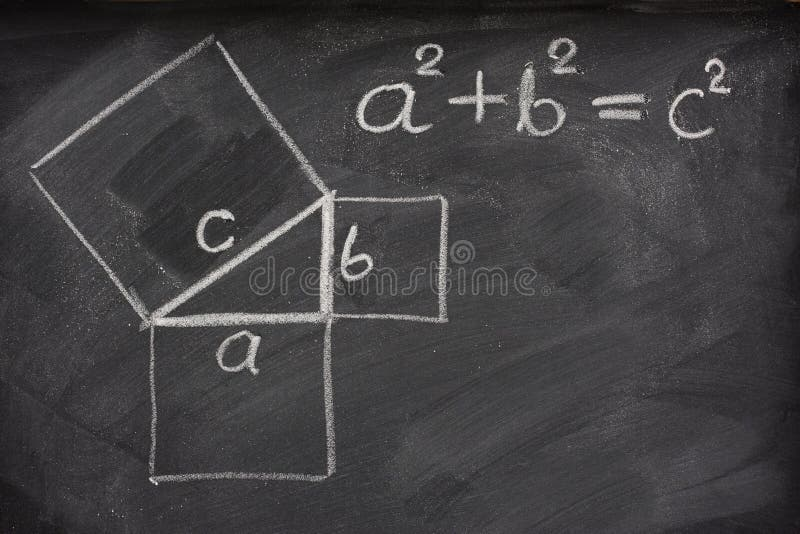Pythagorean theorem on blackboard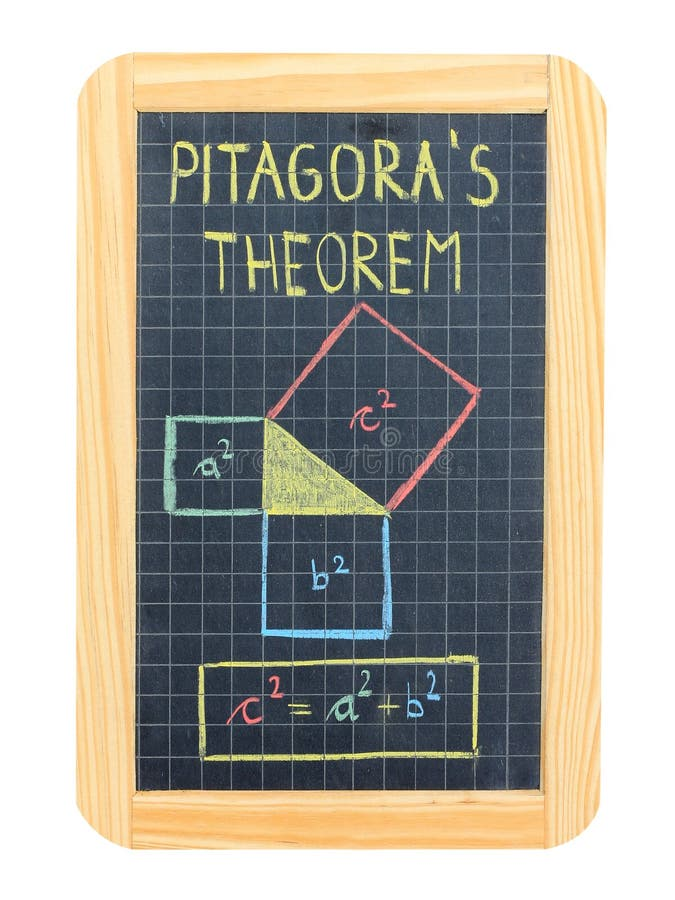Pythagorean theorem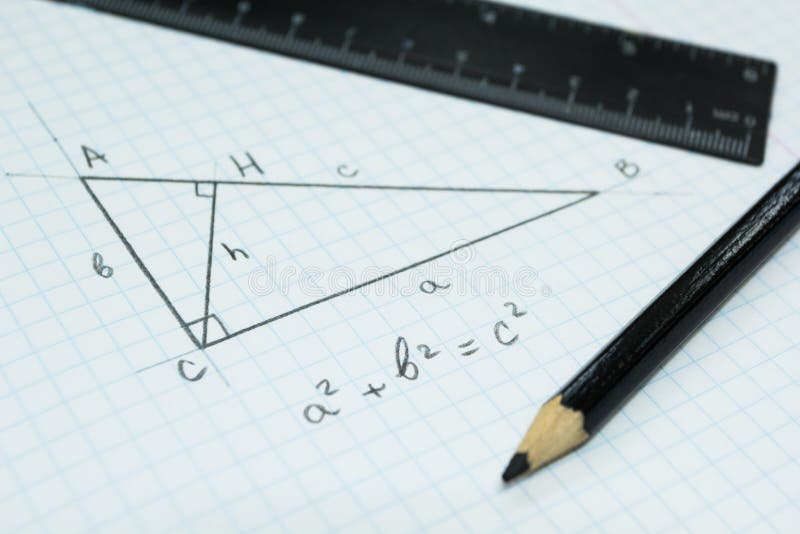Geometry, the Pythagorean theorem in a school notebook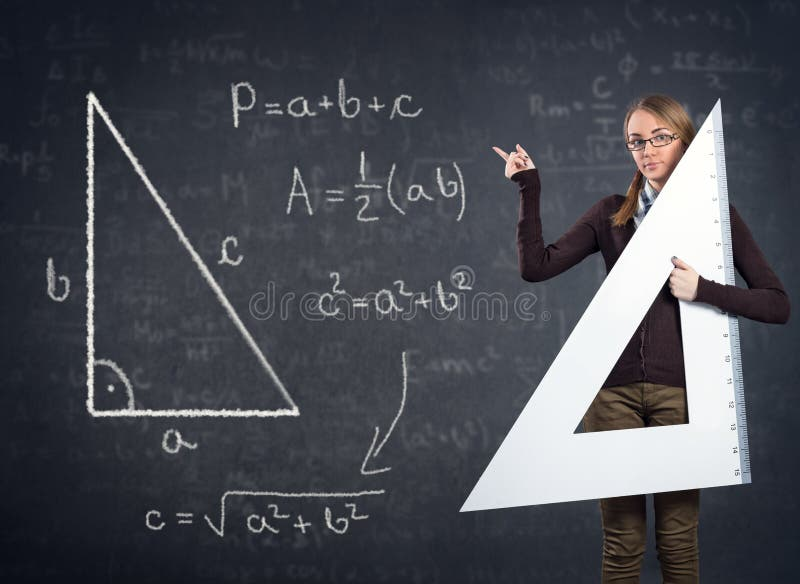Student with a big ruler and Pythagorean theorem on blackboardGraphical demonstration of the Pythagorean Theorem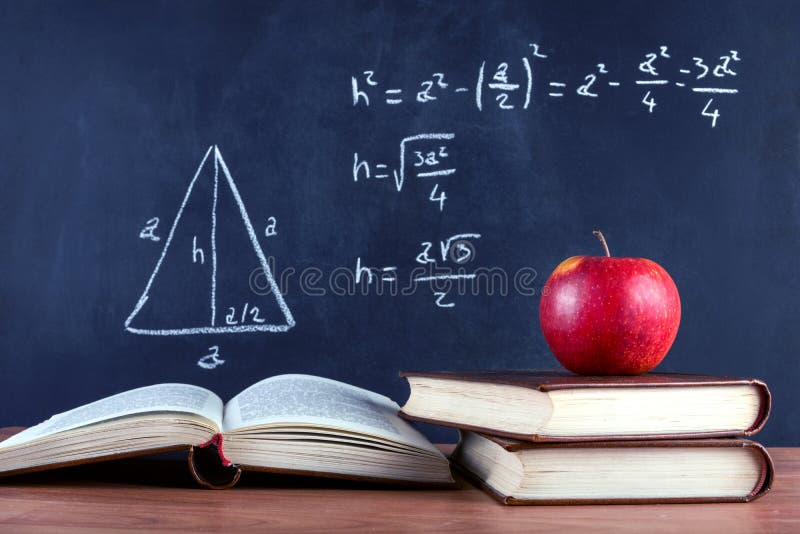Apple on books and blackboard with Pythagorean theorem
More stock photos from Igor Stevanovic's portfolio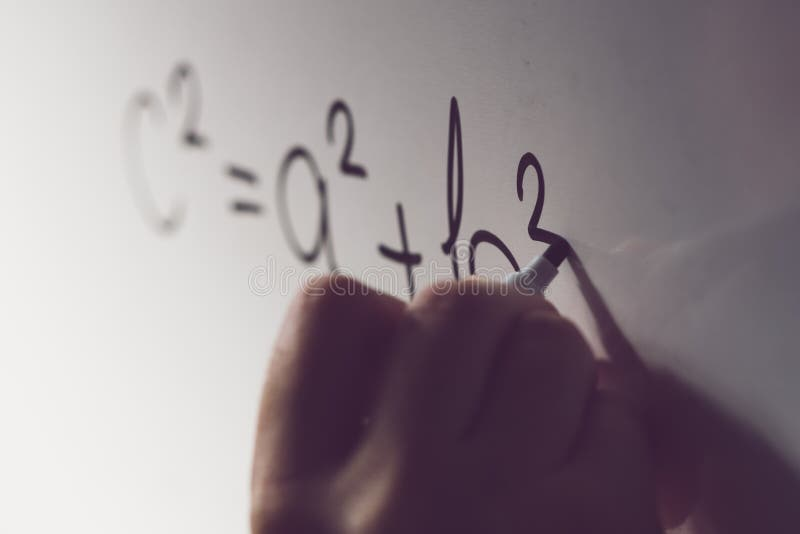Pythagorean theorem, math class in the school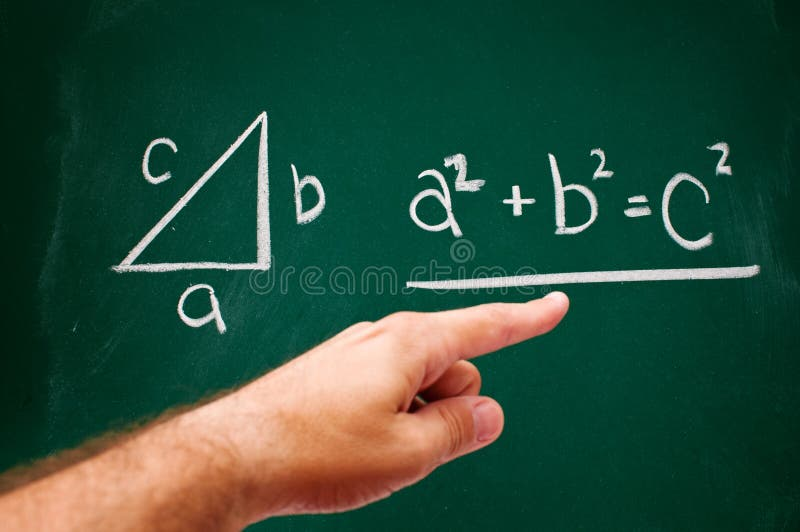Pythagorean theorem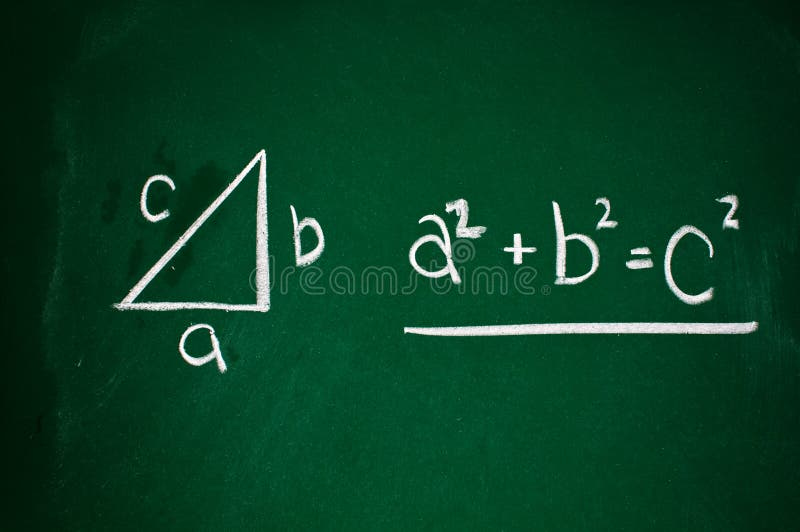Pythagorean theorem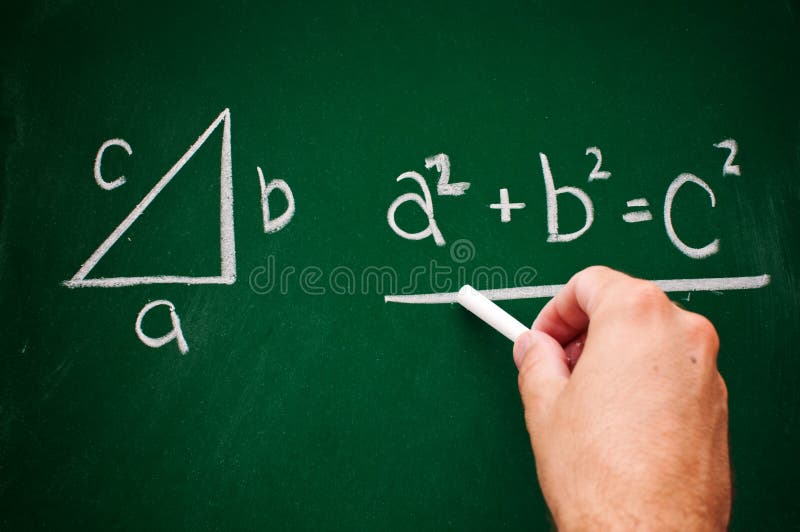Pythagorean theorem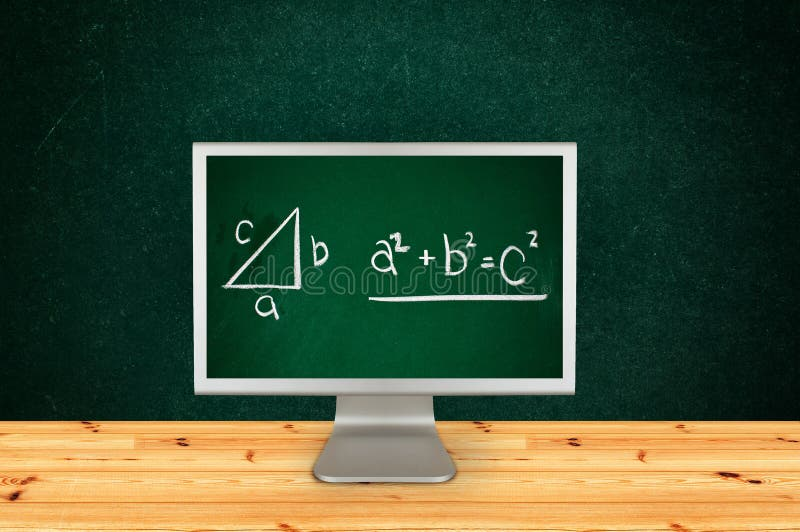Pythagorean theorem
Related categories